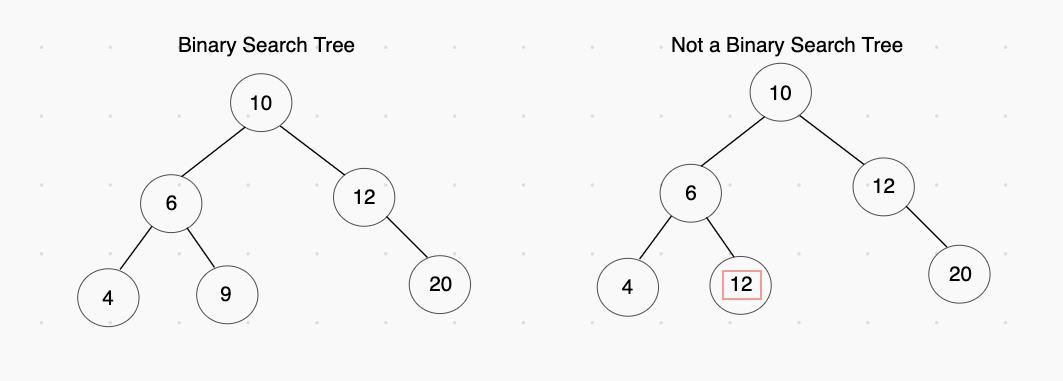# Data Structures & Algorithms 101: Trees/Binary Trees

Welcome back to Data Structures & Algorithms 101, I am glad you can join me on my journey through Data Structures and Algorithms. This week, we’ll be discussing trees! Trees are a non-linear data structures composed of nodes. You might be familiar with binary trees, which are a type of tree, and we will get into those later in this blog. Understanding trees are very important because they are some of the trickiest problems that interviewees face in technical interviews. Since they are not linearly organized, searching through a tree will prove to be much more difficult than searching through an array or linked list. The first thing I want to cover are some of the basic characteristics of trees.

## What Makes a Tree a Tree?

Well to begin, as I stated earlier, a tree is a data structure that is composed of nodes. Although a tree can have any number of nodes, almost every tree has a root node. The root node will have zero or more child nodes and each child node will have zero or more child nodes, and so on. If a node does not have any children, it is called a leaf node. Also, a tree cannot contain cycles and they do not have to be in any particular order.

The way trees are classified are through the number of nodes that each node can hold. For example, if you look at the example below, the root node with a value of 10, has 3 child nodes. This would be considered a ternary tree because a node is able to have three (ternary) child nodes.

However, the most common tree you will see and probably encounter in an interview are binary trees. You have probably heard of binary tress before, but if not, you might be able to deduce that unlike the ternary tree above, binary trees have nodes that hold up to two child nodes. Now, the most common binary trees you will most likely encounter are binary search trees. The difference between any old binary tree and a binary search tree is that there is a certain order that the binary search tree must follow. For a binary search tree, all left descendants of a node must be less than or equal to the value of the node. All right descendants or children of a node must be greater than or equal to the value of the node. Look at the example below to see the difference between the two.Example of the difference between a binary tree and a binary search tree

If you look at the example above, in the binary search tree, you will see that for each child node, the left descendants are always less than the parent nodes and the right descendants are always more than the parent. However, when you look at the binary tree that is not a binary search tree, although the node with value 12 (squared in red) is greater than its parent node value of 6, it is also greater than the value of the root node of 10. Since it is a left descendant of the root node, it does not follow the order of a binary search tree and is not considered one. You can see from this example that the order must be followed by ALL node descendants and not just its immediate children.

## Three Forms of a Binary Tree

There are a multitude of different types of binary trees in data structures and algorithms, including red-black trees, AVL trees, etc., but I’m only going to go over three different forms that binary trees can exhibit.

The first is the Complete Binary Tree, which is a binary tree in which every level of the tree is fully filled. However, the last level does not have to be fully filled, but if the last level is filled it is filled from left to right.

As you can see from the example above, since the binary tree on the right is filled from the left, it is a complete binary tree while the binary tree on the left is incomplete.

The second form is a Full Binary Tree, which is a tree where every node has either zero (0) or two (2) children. None of the nodes in a full binary tree will have one child.

From the example above, the binary tree on the left has a node that has only one child node, which does not make it a full binary tree. However, the binary tree on the right has all of its nodes with either 0 or 2 child nodes, which fulfills the requirements of being considered a full binary tree.

The last form I want to discuss is the Perfect Binary Tree. The perfect binary tree is one where all interior nodes have two (2) child nodes and all leaf nodes are at the same level. Below is an example of a perfect binary tree, although it can also be much larger or much smaller.